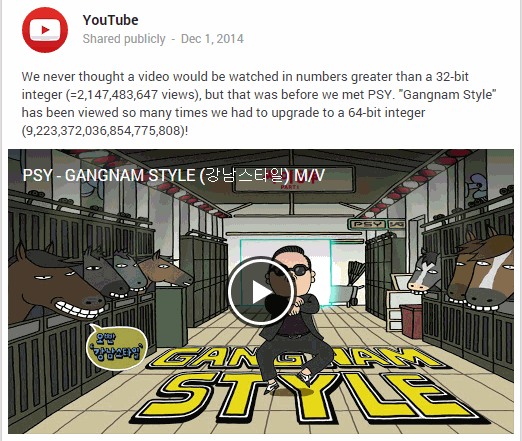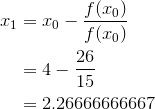Search IntMath
Close

# Newton’s Method, accuracy and floating point numbers

By Murray Bourne, 30 Jul 2015

I recently added a new graph applet which helps you explore the algorithm used in Newton's Method.

## Problems with computer numbers

It's likely you've seen Psy's Gangnam Style video, since it's been watched over 2 billion times.

The number 2 billion is significant because the YouTube engineers had to change the integer type for their viewer number system as a result.

Here's a post they wrote on Google+ at the time:In fact, the number 2,147,483,647 is important in computer programming, because it's the limit of what are called 32-bit integers. YouTube could see they were going to have a problem as the number of views of Psy's video approached the 2 billion mark.

Why is 2.1 billion a limit?

The larger your numbers, of course, it takes more storage in databases, and more computer memory when manipulating those numbers.

Also, YouTube started in the days when 32-bit computer architecture was common. This meant that 32-bit numbers (the ones with an upper limit of 2 billion) were relatively efficiently handled in less steps, and with less memory, than 64 bit numbers.

Now that 64-bit is the standard, it was logical for YouTube to change the integer type for their viewer counter to 64-bit. It meant that any large number would be more correctly represented. So now, any YouTube video can be watched up to 9,223,372,036,854,775,807 times, the highest possible value with a 64-bit number. Time will tell if this will ever be breached.

## Odd things happen near the limits

In the article Floating Point Numbers Aren't Real, (part of the series (97 Things Every Programmer Should Know), we learn that odd things happen when we use computers to perform simple arithmetic near the cut-off value for a 32-bit integer.

For example, if we subtract 64 from this number, its value is unchanged. If we subtract 65 from the number, its value drops 127 places. This is because the spacing between adjacent floating point numbes in that range is 128, and rounding means the displayed value is the nearest floating point number.

Another oddity is the following.

Let's add 0.7 and 0.1, then multiply by 10 and round down the result.

Our code is something like: floor((0.7 + 0.1)*10)

We should get 8, right? But in fact, the computer outputs 7.

This is because the internal representation of (0.7 + 0.1)*10 is something like 7.99999999984.

When we find the nearest integer below that value, of course, it's 7.

I came across some of these issues when developing the Newton's Method graph applet, as explained next.

### Newton's Method computer inaccuracies

In the new interactive graph, you'll notice in the first example presented, f(x) = 2x2x − 2, that the first few approximations are as follows:

x0 = 4.00000000000000

x1 = 2.26666666662157

x2 = 1.52176308539695

x3 = 1.30360871696562

x4 = 1.28102380109538

But in this example,

f(x) = 2x2x − 2, so f(4) = 32 − 4 − 2 = 26,

and

f'(x) = 4x − 1, so f'(4) = 4(4) − 1 = 15.

Substituting in the formula for Newton's Method, our next approximation should be:However, the graph applet has 2.26666666662157. Why the discrepancy at the end?

This is a trap in all numerical computing. I'm using javascript, and internally, the computer is using floating point numbers.

When our numbers are very small (like in my example) or very large (as in the case of Gangnam Style), there will always be inaccuracies due to floating point numbers. We should always build in error checking, and if the error is beyond what is acceptable, we need to implement an algorithm to correct for it.

Most computer algorithms (used by Matlab, Mathematica and so on), have such checking algorithms and so generally produce a better result.

## Conclusion

It's always good to be aware of how computers represent numbers. There are times when we get unexpected results, and we need to know the limitations of the algorithm we are using.

Be the first to comment below.

### Comment Preview

HTML: You can use simple tags like <b>, <a href="...">, etc.

To enter math, you can can either:

1. Use simple calculator-like input in the following format (surround your math in backticks, or qq on tablet or phone):
a^2 = sqrt(b^2 + c^2)
(See more on ASCIIMath syntax); or
2. Use simple LaTeX in the following format. Surround your math with $$ and $$.
$$\int g dx = \sqrt{\frac{a}{b}}$$
(This is standard simple LaTeX.)

NOTE: You can mix both types of math entry in your comment.